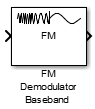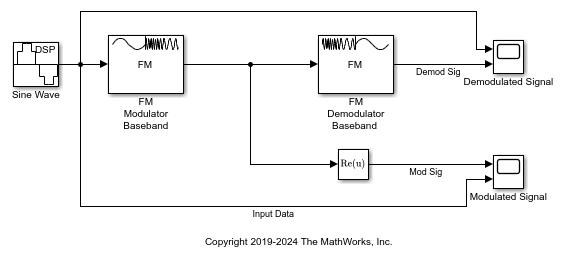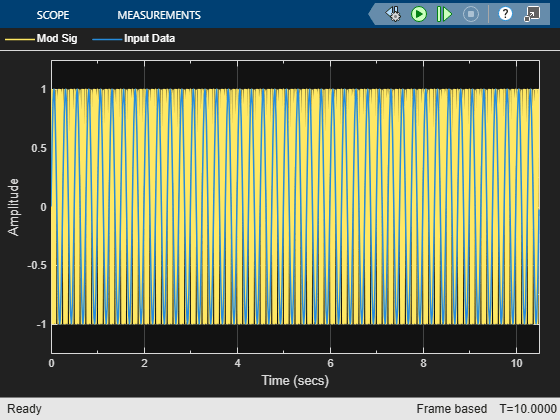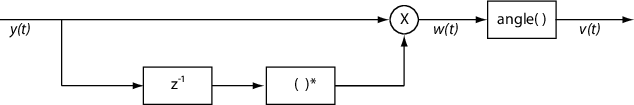# FM Demodulator Baseband

Demodulate using FM method

•Libraries:
Communications Toolbox / Modulation / Analog Baseband Modulation

## Description

The FM Demodulator Baseband block demodulates a complex input signal and returns a real output signal.

## Examples

expand all

Modulate and demodulate a sinusoidal signal using FM Modulator Baseband and FM Demodulator Baseband blocks.

The `fmmoddemod` model generates a sine wave of frequency 4 Hz and amplitude 1 V. The FM Modulator Baseband block sets the frequency deviation to 50 Hz.The Modulated Signal scope shows that the frequency of the modulator output, `Mod Sig`, varies with the amplitude of the input data.The Demodulated Signal scope demonstrates that the output of the demodulator, `Demod Sig`, is perfectly aligned with the input data.## Ports

### Input

expand all

Input signal, specified as a real scalar, vector, or matrix.

Data Types: `double` | `single`

### Output

expand all

Output signal, returned as a real scalar, vector, or matrix. The data at this port has the same data type and size as the input signal.

Data Types: `double` | `single`

## Parameters

expand all

To edit block parameters interactively, use the Property Inspector. From the Simulink® Toolstrip, on the Simulation tab, in the Prepare gallery, select .

Frequency deviation of the demodulator, in Hz, specified as a positive scalar. The system bandwidth is equal to twice the sum of the frequency deviation and the message bandwidth.

Type of simulation to run, specified as `Code generation` or `Interpreted execution`.

• `Code generation` — Simulate the model by using generated C code. The first time you run a simulation, Simulink generates C code for the block. The model reuses the C code for subsequent simulations unless the model changes. This option requires additional startup time, but the speed of the subsequent simulations is faster than with the `Interpreted execution` option.

• `Interpreted execution` — Simulate the model by using the MATLAB® interpreter. This option shortens startup time, but the speed of subsequent simulations is slower than with the ```Code generation``` option. In this mode, you can debug the source code of the block.

## Block Characteristics

 Data Types `double` | `single` Multidimensional Signals `no` Variable-Size Signals `no`

## Algorithms

A frequency-modulated passband signal, Y(t), is given as

`$Y\left(t\right)=A\mathrm{cos}\left(2\pi {f}_{\text{c}}t+2\pi {f}_{\text{Δ}}{\int }_{0}^{t}x\left(\text{τ}\right)d\text{τ}\right)\text{\hspace{0.17em}},$`

where:

• A is the carrier amplitude.

• fc is the carrier frequency.

• x(τ) is the baseband input signal.

• fΔ is the frequency deviation in Hz.

The frequency deviation is the maximum shift from fc in one direction, assuming |x(τ)| ≤ 1.

A baseband FM signal can be derived from the passband representation by downconverting the passband signal by fc such that

`$\begin{array}{c}{y}_{\text{s}}\left(t\right)=Y\left(t\right){e}^{-j2\pi {f}_{\text{c}}t}=\frac{A}{2}\left[{e}^{j\left(2\text{π}{f}_{\text{c}}t+2\text{π}{f}_{\text{Δ}}{\int }_{0}^{t}x\left(\text{τ}\right)d\text{τ}\right)}+{e}^{-j\left(2\text{π}{f}_{\text{c}}t+2\text{π}{f}_{\text{Δ}}{\int }_{0}^{t}x\left(\text{τ}\right)d\text{τ}\right)}\right]{e}^{-j2\text{π}{f}_{\text{c}}t}\\ =\frac{A}{2}\left[{e}^{j2\text{π}{f}_{\text{Δ}}{\int }_{0}^{t}x\left(\text{τ}\right)d\text{τ}}+{e}^{-j4\text{π}{f}_{\text{c}}t-j2\text{π}{f}_{\text{Δ}}{\int }_{0}^{t}x\left(\text{τ}\right)d\text{τ}}\right]\text{\hspace{0.17em}}.\end{array}$`

Removing the component at -2fc from yS(t) leaves the baseband signal representation, y(t), which is given as

`$y\left(t\right)=\frac{A}{2}{e}^{j2\pi {f}_{\Delta }{\int }_{0}^{t}x\left(\tau \right)d\tau }.$`

The expression for y(t) can be rewritten as $y\left(t\right)=\frac{A}{2}{e}^{j\varphi \left(t\right)}$, where $\varphi \left(t\right)=2\text{π}{f}_{\Delta }{\int }_{0}^{t}x\left(\tau \right)d\tau$. Expressing y(t) this way implies that the input signal is a scaled version of the derivative of the phase, ϕ(t).

To recover the input signal from y(t), use a baseband delay demodulator, as this figure shows.Hatai, I., and I. Chakrabarti. “A New High-Performance Digital FM Modulator and Demodulator for Software-Defined Radio and Its FPGA Implementation.” International Journal of Reconfigurable Computing (December 25, 2011): 1–10. https://doi.org/10.1155/2011/342532.

 Taub, H., and D. Schilling. Principles of Communication Systems. McGraw-Hill Series in Electrical Engineering. New York: McGraw-Hill, 1971, pp. 142–155.